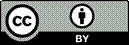Article citation information:

Pawelski, Z., Zdziennicki, Z. Determining the torque loading of the cycloid gear. Scientific Journal of Silesian University of Technology. Series Transport. 2018, 101, 131-140. ISSN: 0209-3324. DOI: https://doi.org/10.20858/sjsutst.2018.101.12.

Zbigniew PAWELSKI, Zbigniew ZDZIENNICKI

Summary. Based on experimental investigations, a very close correlation was established between the torque loading of the cycloid gear and the laser sensor’s indications measuring the displacement of the gear body. The method is given and the relationship between the registered signal of the torque loading of the gear and the registered laser sensor signal is determined. The practical application of this property in the industrial diagnostics of cycloidal gears is discussed. Spectral analysis of the fast Fourier transform (FFT) signal ripple from a laser sensor is made. These results are compared with the results of the analysis carried out on the ripples of the torques and rotational speeds of the cycloid gear. This comparison shows that the spectral FFT analysis of the ripples of the laser sensor signal is a useful tool in the diagnostics of cycloidal gears.

Keywords: cycloidal gear; load torque; laser signal; FFT analysis

1. INTRODUCTION

The test object and test stand are described in . The test stand has been additionally equipped with a laser sensor for measuring the horizontal displacement of the cycloidal gear housing (see Figure 1).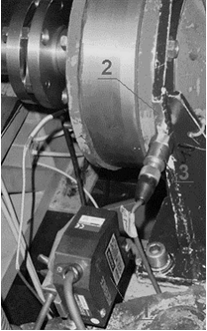Fig. 1. Laser sensor on the test bench:

1) laser sensor, 2) the surface on which displacement is measured by the laser sensor,

3) piezoelectric sensor

Characteristic features of the laser sensor are as follows:

a.       Manufacturer: Keyence Corporation

b.      Sensor model: LK-K157

c.       Controller model: LK-G5001

The purpose of the analysis is to check the possibility of determining the property and size of the load torque by means of the laser sensor signal. The test conditions are given below:

Tested object: Three-disc Cyclo gearbox (prototype)

Work mode: Reducer

Input speed (mean): 1,600 rpm

Output torque (mean): 495 Nm

Type of oil: ATF M3 Plus

Temperature of oil: 37.4°C

2. MEASURED SIGNALS

The form of the measured and recorded signals is shown in Figure 2. This figure shows a very large similarity between the output torque of the cycloidal gear (load) and the horizontal displacement of its housing (signal from the laser sensor).

This similarity prompted the question about whether the torque of the cycloidal gear load can be estimated by means of a laser signal for measuring the horizontal displacement of the gear housing.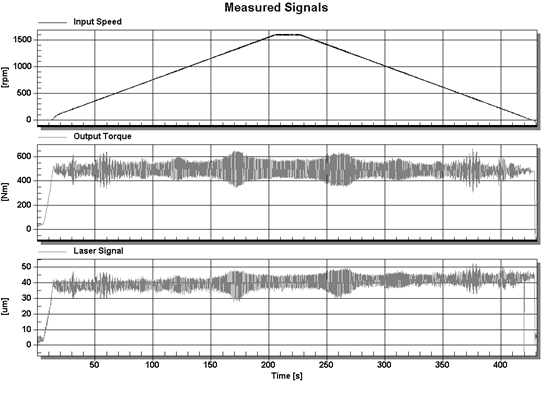Fig. 2. Measured and recorded signals

The first step was to determine the static characteristics of the mean value of the laser signal as a function of the average torque load of the cycloid gear. The results of these calculations are presented in Figure 3.

As can be seen from the characteristics set out in Figure 3, the correlation coefficients between the analysed values are very high. This motivated us to analyse the dynamic relations between the torque signal of the load and the laser signal measuring the horizontal deflections of the cycloidal gear.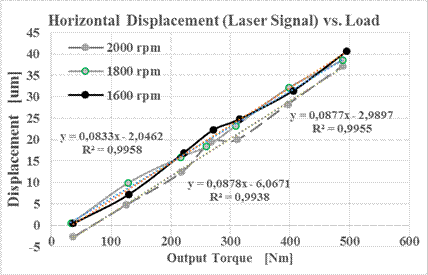Fig. 3. Static characteristics of the laser signal as a function of the output torque

3. ANALYSED SIGNALS

The analysis was carried out for half of the recorded signals, i.e., when the value of the rotational speed of the cycloidal gearbox increases and remains constant. It is possible to observe a large similarity in the course of changes in the output torque signal along with the signal from the laser sensor. This applies to both the time and input speed functions. The latter are of more practical significance. (Figures 4-5).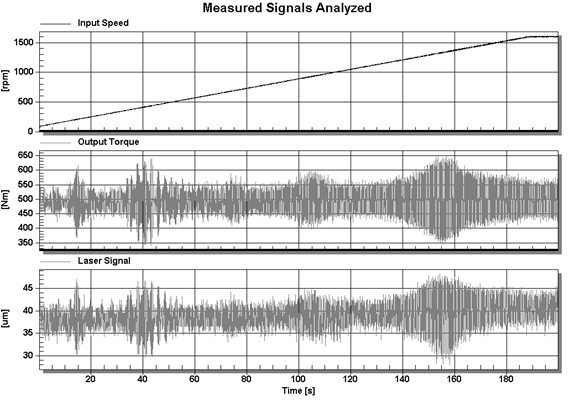Fig. 4. Analysed signals as a function of time

As can be easily noticed, the laser signal course accurately reflects the rotational speed zone in which the output torque of the cycloidal gear increases.

In Figure 5, characteristic revolutions can be distinguished: [40, 290, 550, 800, 1,200 and 1,600 (idem)] rpm. They correspond to amplitudes: ±(110, 150, 70, 100, 150 and 60) Nm and ±(8, 9, 5, 6, 10 and 4) μm. The reaction torque of the gear housing is the sum of the torque on the input and output shaft; hence, the average value of the vibration recorded by the laser sensor increases with the revolutions as opposed to the torque at the exit from the transmission. In addition, the character of changes in both parameters is almost identical.

By entering the conversion factor: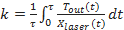(1)

where: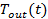- torque on the output shaft in Nm,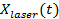- horizontal displacement of the gear housing in μm.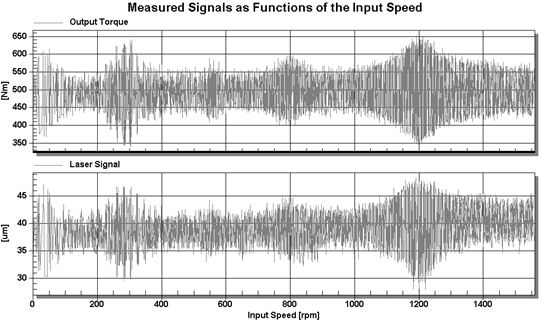Fig. 5. Comparison of both torques as function of input speed

The compatibility of both signals is obtained as in Figure 6.

The analysis of the tested signals was carried out using the CatMan program. This useful, powerful tool enables the processing of digital signals containing hundreds of thousands of measurement points. A screenshot of this program for the analysed signals is shown in Figure 5. In order to represent the torque of the cycloidal gear load by a laser signal measuring the horizontal displacement of its housing, the appropriate coefficient should be determined.

The multiplication factor was determined by dividing the output torque by the laser signal as the mean value of the new variable obtained (Figures 5-6). This factor equalled 12.69.

The comparison of the output torque with the torque calculated from the laser signal (multiplication factor=12.69) is shown in Figure 5 (as a function of time) and Figure 6 (as functions of the input speed).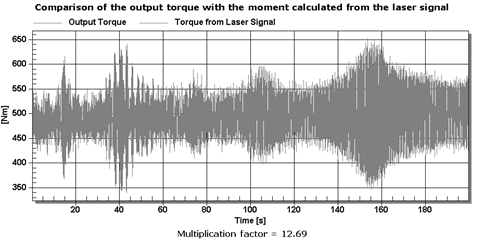Fig. 6. Comparison of both torques as a function of time for the factor k=12.69

For the coefficient of k=13, a comparison of these signal runs is shown in Figures 7-8.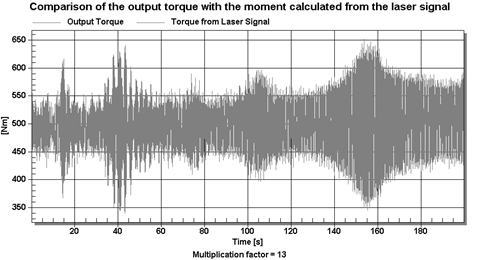Fig. 7. Comparison of both torques as a function of time for the factor k=13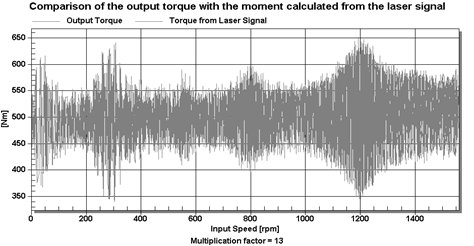Fig. 8. Comparison of both torques as a function of input speed for the factor k=13

As for the average value for these runs, it is practically the same (coefficient k=12.69). However, the maximum value is 6.5% lower for the moment calculated from the laser signal (Figures 5 and 6).

For the factor k=13, the mean values of the torques differ by 2.7% (the calculated moment for laser is higher) and the maximum amplitude for the laser torques is 9.8% higher (Figures 7-8). Thus, the correction increases errors.

3. FFT ANALYSIS

Time curves of the analysed signals have been subjected to FFT analysis. The results of the FFT analysis are presented in Figure 9.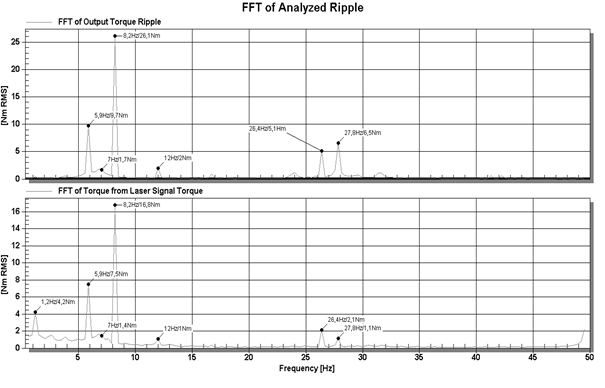Figure 9. The results of the FFT analysis

The FFT analysis parameters are as follows: the number of discrete values=4,096, sampling (or capture) frequency=1,200 Hz for output torques and laser signal. Thus, the spectrum frequency range is 0÷600 Hz, while the frequency resolution (spacing between frequency lines) is 0.29 Hz.

As shown in Figure 10, in the range up to 50 Hz, almost full compliance of the measurements was obtained from the torque and laser sensor. The frequencies are identical and the amplitudes are similar. In addition, the frequencies from Figure 10 are similar to those in Figure 5, with their comparison shown in Table 1.Fig. 10. The results of the FFT analysis at 1,200 rpm

As shown, for example, in Figure 10, above 30 Hz, there are higher harmonic vibrations: meshing and fundamental input shaft.

Table 1 lists the FFT frequencies for characteristic speeds: (40, 290, 550, 800 and 1,200) rpm during the acceleration phase and fixed at 1,600 rpm, with a torque at the output shaft of 495 Nm. The aforementioned speeds are approximate, due to the variable acceleration phase process; hence, the different values read in the course of FFT analysis.

Taking into account k=12.69 Nm/μm, the amplitudes from the laser sensor were calculated according to the readings from the torque counter. The results from the torque sensor are highlighted in yellow. In addition, the results for a fixed rotational speed of 1,200 rpm of the input shaft are provided.

Table 1

List of significant frequencies

 rpm I II III IV V 42 35 1.0 Hz/1.8 5.1 Hz/2.1 310 278 1.2 Hz/3.5 1.2 Hz/3.5 3 Hz/2.4 3 Hz/2.4 6.7 Hz/20.7 5.7 Hz/20.7 446 418 2.6 Hz/2.8 2.6 Hz/2.8 6.7 Hz/34.3 6.7 Hz/24.1 13.8 Hz/2.6 13.8 Hz/1 546 523 3.5 Hz/2.6 3.5Hz/2.3 5 Hz/7 5 Hz/5.5 7 Hz/17.9 7 Hz/12 11.4 Hz/6.6 11.4 Hz/2.1 23.7 Hz/1.7 804 783 4.7 Hz/6.3 4.7Hz/5.0 6.7 Hz/23 6.7 Hz/16 15.5 Hz/3.9 1,200 =idem 4.4 Hz/3.0 4.4 Hz/2.0 6.2 Hz/30.0 6.2 Hz/20.7 8.8 Hz/4.7 8.8 Hz/2.7 12.3 Hz/1.3 19.6 Hz/4.5 20.8 Hz/6.9 1,224 1,179 6.7 Hz/47.7 6.7 Hz/33.4 22.3 Hz/2.9 1,600 =idem 1.2 Hz/4.2 5.9 Hz/9.7 5.9 Hz/7.5 7 Hz/1.7 7 Hz/1.4 8.2 Hz/26.1 8.2 Hz/16.8 12 Hz/2 12 Hz/1 26.4 Hz/5.1 26.4 Hz/2.1 27.8 Hz/6.5 27.8 Hz/1.1

The resonant frequencies set during FFT are characterized by a high conformity of parameters, which allows us to assign them to the characteristic ranges of rotational speeds in Figures 4-6. The frequencies in Column II are characterized by high amplitude (resonance of 550 rpm); in Columns IV and V, there are two very similar parameters, which, when adding up, give a resonance of around 1,200 rpm. A comparison of significant frequencies for 1,600 rpm and various load torques is shown in the table below. Columns with the largest amplitudes are highlighted in yellow.

Table 2

List of significant frequency parameters

 Hz 1.172 2.637 5.859 8.203 12.01 25.2 26.37 27.83 32.81 FFT Input Speed _37Nm 0.0374 0.0281 0.4878 2.16 0.0173 0.6629 0.6463 0.2759 0.4586 FFT Input Speed _130Nm 0.0196 0.018 1.183 2.004 0.0135 0.4305 0.4682 0.1728 0.1 FFT Input Speed _222Nm 0.0211 0.0280 1.209 2.093 0.0260 0.3251 0.3045 0.1334 0.207 FFT Input Speed _272Nm 0.1164 0.0176 0.9384 2.132 0.0184 0.26 0.4191 0.0605 0.1266 FFT Input Speed _316Nm 0.1363 0.0194 0.6705 2.194 0.0220 0.2764 0.3545 0.0514 0.1257 FFT Input Speed _406Nm 0.0613 0.0171 0.4482 1.983 0.0287 0.2125 0.1844 0.0874 0.1065 FFT Input Speed _495Nm 0.0656 0.0152 0.4405 2.106 0.0393 0.1587 0.1752 0.0758 0.1013 FFT Output Speed_37Nm 0.0294 0.020 0.1288 0.0219 0.0229 0.0103 0.1195 0.0765 0.0079 FFT Output Speed_130Nm 0.0316 0.0162 0.1242 0.0129 0.0128 0.0056 0.0767 0.0331 0 FFT Output Speed_222Nm 0.0331 0.0161 0.0959 0.0315 0.0152 0.0038 0.0718 0.0271 0.003 FFT Output Speed_272Nm 0.0311 0.0156 0.0754 0.0407 0.0149 0.0032 0.0725 0.0270 0.0018 FFT Output Speed_316Nm 0.0314 0.0152 0.0573 0.0520 0.0149 0.0033 0.0728 0.0216 0.0014 FFT Output Speed_406Nm 0.0323 0.0159 0.0384 0.0540 0.0164 0.0024 0.0801 0.0216 0.0024 FFT Output Speed_495Nm 0.0338 0.0155 0.0377 0.0623 0.0173 0.0019 0.0833 0.0208 0.0019 FFT Input Torque_37Nm 0.0147 0.0168 0.6309 0.0700 0.0566 0.1979 0.1689 0.2423 0.2032 FFT Input Torque_130Nm 0.0113 0.0150 1.407 0.4636 0.0234 0.1083 0.139 0.2971 0.000 FFT Input Torque_222Nm 0.0106 0.017 1.391 0.791 0.0872 0.0770 0.0810 0.3382 0.0481 FFT Input Torque_272Nm 0.0272 0.0131 1.074 0.9186 0.0789 0.0668 0.1731 0.3745 0.0575 FFT Input Torque_316Nm 0.0320 0.0088 0.757 1.043 0.0837 0.0668 0.1556 0.3435 0.0482 FFT Input Torque_406Nm 0.0196 0 0.5115 1.056 0.0959 0.0479 0.1241 0.3574 0.0395 FFT Input Torque_495Nm 0.0227 0.0114 0.4927 1.23 0.1065 0.0553 0.1604 0.3831 0.0425 FFT Output Torque_37Nm 0.4127 0.3172 12.1 5.293 1.094 0.4709 3.393 2.57 0.2327 FFT Output Torque_130Nm 0.3043 0.2977 27.42 12.37 0.438 0.6719 4.329 3.977 0.000 FFT Output Torque_222Nm 0.2979 0.3188 27.2 18.74 1.661 0.6802 4.706 5.27 0.293 FFT Output Torque_272Nm 0.4345 0.2416 20.78 20.95 1.442 0.6071 5.117 5.728 0.1911 FFT Output Torque_316Nm 0.5806 0.1966 14.59 23.22 1.597 0.5997 5.101 5.325 0.2532 FFT Output Torque_406Nm 0.4237 0.1728 9.868 23.04 1.833 0.6088 5.347 6.153 0.2546 FFT Output Torque_495Nm 0.4093 0.1939 9.326 26.01 1.998 0.3874 5.133 6.554 0.2871 FFT of Laser Signal_37Nm 2.659 1.047 8.966 3.148 0.512 0.165 1.784 0.356 0.140 FFT of Laser Signal_130Nm 3.061 0.854 20.32 7.953 0.390 0.308 1.714 0.490 0.000 FFT of Laser Signal_222Nm 3.578 0.857 21.06 12.37 0.449 0.295 1.791 0.839 0.184 FFT of Laser Signal_272Nm 3.186 1.445 16.20 13.99 0.640 0.248 2.474 1.263 0.343 FFT of Laser Signal_316Nm 3.300 1.643 11.65 15.74 0.908 0.261 2.143 1.103 0.227 FFT of Laser Signal_406Nm 3.340 1.918 7.607 14.51 0.564 0.242 1.814 0.979 0.228 FFT of Laser Signal_495Nm 4.019 1.567 7.502 16.77 1.081 0.237 2.111 1.113 0.205

According to the literature [2,3,5,7], the rotational speed of the input shaft, i.e., 1,600 rpm, corresponds to fundamental frequencies of 1.4 Hz and 26.7 Hz and the frequency of meshing, 28.1 Hz. The results in the above table show a high compliance with the theory, but other characteristic values also occur.

5. CONCLUSIONS

The torque calculated from the laser sensor signal can be an interesting diagnostic signal. It is possible to estimate the load torque of the cycloidal gear on this basis.

Analysis of this signal can be a good alternative for the analysis of signals from piezoelectric sensors in the low-frequency range.

This paper contains the first step in discussing this issue.

References

1.             Bao J., W. He. 2015. “Parametric Design and Efficiency Analysis of the Output-pin-wheel Cycloid Transmission”. International Journal of Control and Automation 8(8): 349-362.

2.             Cochran V., T. Bobak. 2008. A Methodology for Identifying Defective Cycloidal Reduction Components Using Vibration Analysis and Techniques. AGMA Technical Paper 08FTM02.

3.             Gaidys R., D. Zizys, R. Dauksevicius, V. Ostaševičius. 2013. “Segmentation of piezoelectric layers based on the numerical study of normal strain distributions in bimorph cantilevers vibrating in the second transverse mode”. Mechanika 4: 451-458.

4.             Lai T., W. Hsieh, G. Chen. 2007. “Geometrical Design of Roller Drives with Two-tooth Difference”. Journal of the Chinese Society of Mechanical Engineers 28(6): 641-648.

5.             Naginevicius V., V. Ostasevicius, A. Bubulis, A. Palevicius. 2015. “Vibration assisted spring loaded micro spray system for biomedical application”. Mechanika 4: 285-289.

6.             Pawelski Z., Z. Zdziennicki. 2018. “FFT Analysis of Torque and Speed Ripples as a Diagnostic Tool for a Cycloidal Gear”. Journal of Measurements in Engineering (in press).

7.             Plugin A., L. Trykoz, O. Herasymenko, A. Pluhin, V. Konev. 2018. “Independent diagnostic computer systems with the ability to restore operational characteristics of construction facilities”. Diagnostyka 19(2): 11-21. DOI: 10.29354/diag/83009.

8.             Warda B. 2006. “Test Rig for Lifetime Testing of Meshing of the Cyclo Gear”. Tribologia 6: 131-140.

9.             Yang B., Y. Liu, G. Ye. 2012. “Research of ArtemiS in a Ring-plate Cycloid Reducer”. Journal of Software 7(12): 2671-2677.

10.         Zah M., D. Lates, V. Csibi. 2012. “Thermal Calculation for Planetary Cycloidal Gears with Bolts. Acta Universitatis Sapientiae Electrical and Mechanical Engineering 4: 103-110.

Received 01.08.2018; accepted in revised form 14.11.2018